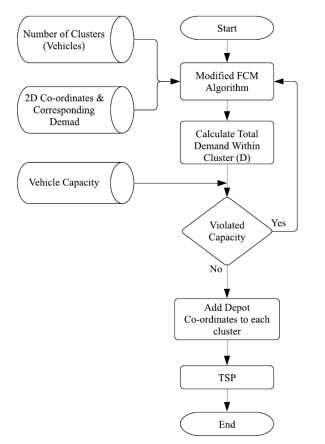Journal

# Supervised fuzzy C-means techniques to solve the capacitated vehicle routing problem

By
Shalaby M.
Mohammed A.
Kassem S.

Fuzzy C-Means (FCM) clustering technique is among the most effective partitional clustering algorithms available in the literature. The Capacitated Vehicle Routing Problem (CVRP) is an important industrial logistics and managerial NP-hard problem. Cluster-First Route-Second Method (CFRS) is one of the efficient techniques used to solve CVRP. In CFRS technique, customers are first divided into clusters in the first phase, then each cluster is solved independently as a Traveling Salesman Problem (TSP) in the second phase. This research is concerned with the clustering phase of CFRS, and TSP is then solved using a traditional optimization method. Three supervised FCM based techniques are proposed to solve the clustering phase at reduced cost via centroids (pre-FCM) initialization phase. The proposed pre-FCM initialization techniques are developed to be problem dependent. Hence, three initialization techniques are first developed using K-means technique, spatially equally distributed, and demand weighted center of mass. Then, a modified demand weighted fuzzy c-means objective function is employed to assign customers to clusters. To compare the performance of the proposed supervised FCM techniques, forty-two CVRP benchmark problems are solved using the traditional fuzzy C-means algorithm and the developed algorithms. Extensive comparisons are conducted between the traditional fuzzy C-means algorithm, the three proposed initialization techniques, and other fuzzy C-means techniques available in the literature. Results show that the proposed three initialization techniques are efficient in terms of solution quality and computational cost. © 2021, Zarka Private University. All rights reserved.